#Calculus Worksheets

## Calculus Worksheets for Practice and Study

Here is a graphic preview for all of the Calculus Worksheets Sections. You can select different variables to customize these Calculus Worksheets for your needs. The Calculus Worksheets are randomly created and will never repeat so you have an endless supply of quality Calculus Worksheets to use in the classroom or at home. These Calculus Worksheets consist of Integration, Differential Equation, Differentiation, and applications Worksheets for your use. Our Calculus Worksheets are free to download, easy to use, and very flexible.

These Calculus Worksheets are a great resource for students in high school.

Click here for a Detailed Description of all the Calculus Worksheet Sections.

## Quick Link for All Calculus Worksheet Sections

Click the image to be taken to that Calculus Worksheet Section.

##### Limits and ContinuityCalculus Worksheets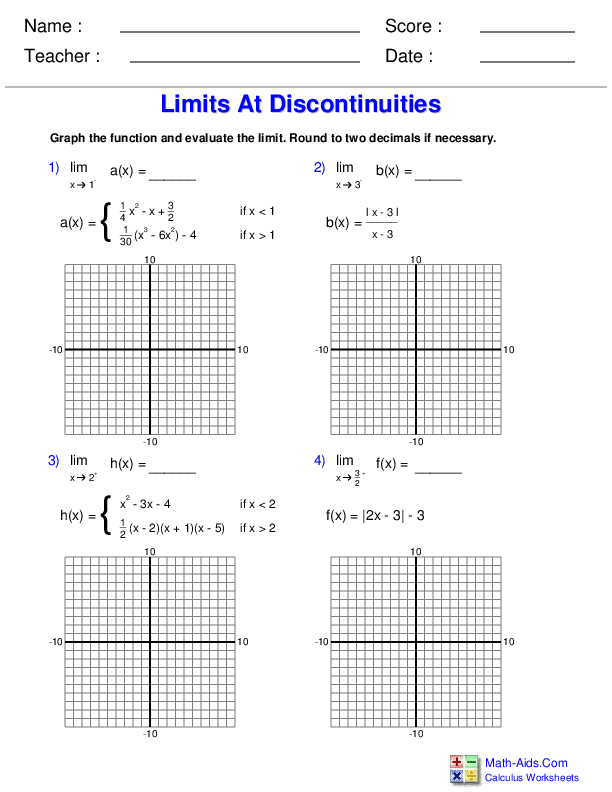##### Differentiation RulesCalculus Worksheets##### Differential EquationsCalculus Worksheets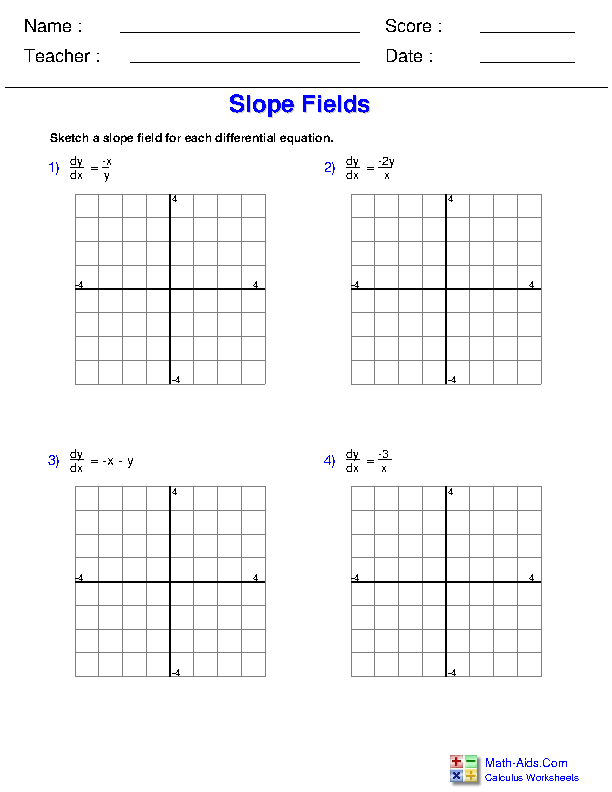##### Differentiation ApplicationsCalculus Worksheets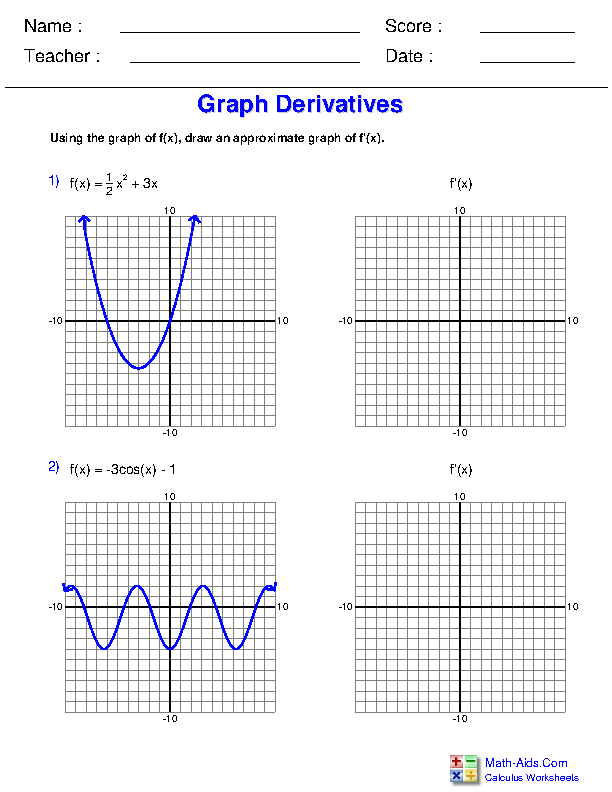##### Definite IntegrationCalculus Worksheets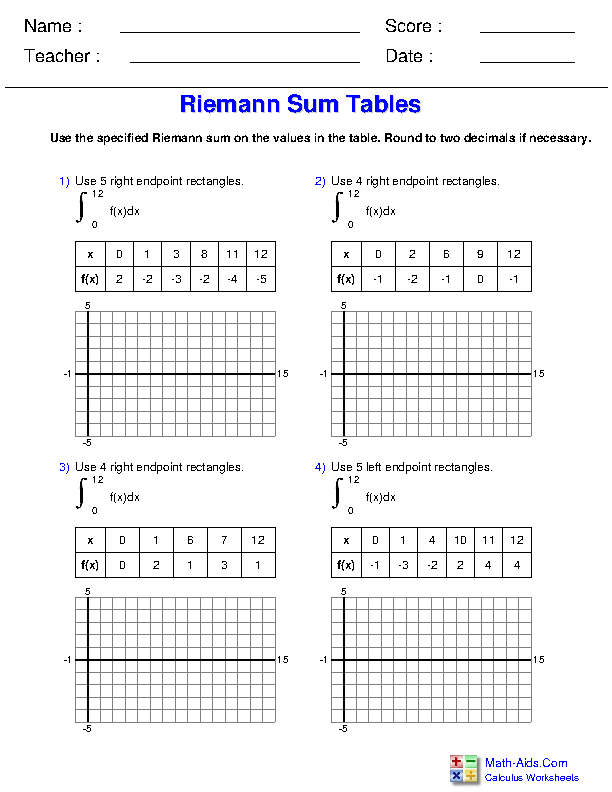##### Indefinite IntegrationCalculus Worksheets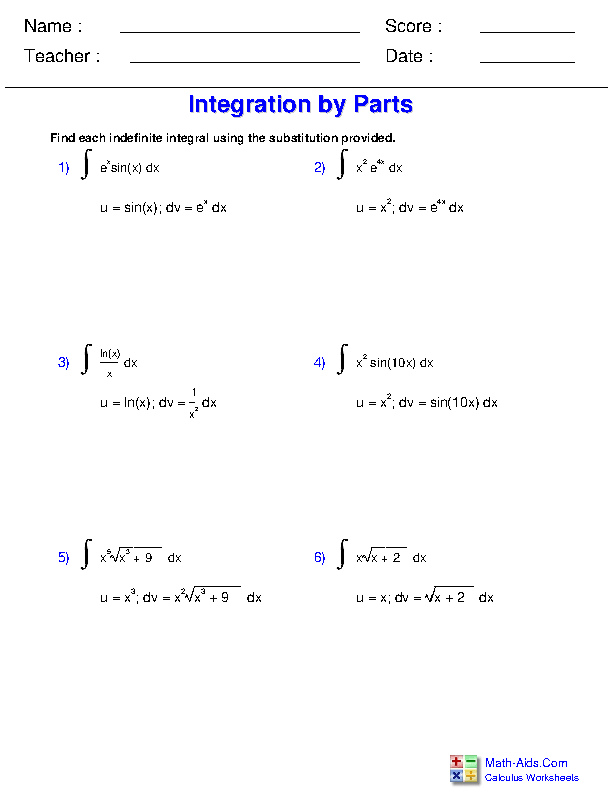##### Integration ApplicationsCalculus Worksheets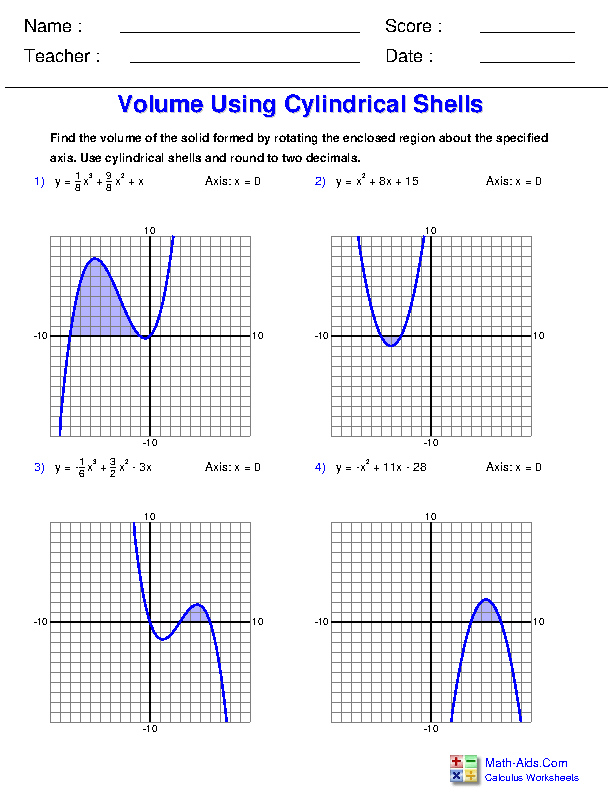Recommended Videos

## Detailed Description for All Calculus Worksheet Sections

Limits and Continuity Worksheets
This section contains all of the graphic previews for the Limits and Continuity Worksheets. We have differentiation tables, rate of change, product rule, quotient rule, chain rule, and derivatives of inverse functions worksheets for your use. These Calculus worksheets are a good resource for students in high school.

Differentiation Rules Worksheets
This section contains all of the graphic previews for the Differentiation Rules Worksheets. We have differentiation tables, rate of change, product rule, quotient rule, chain rule, and derivatives of inverse functions worksheets for your use. These Calculus worksheets are a good resource for students in high school.

Differential Equations Worksheets
This section contains all of the graphic previews for the Differential Equations Worksheets. We have slope field, separable equation, differential equation, and exponential growth worksheets for your use. These Calculus worksheets are a good resource for students in high school.

Differentiation Applications Worksheets
This section contains all of the graphic previews for the Differentiation Applications Worksheets. We have rate of change, graphing, graph properties, differentials, optimization, Newton's Method, and related rates worksheets for your use. These Calculus worksheets are a good resource for students in high school.

Definite Integration Worksheets
This section contains all of the graphic previews for the Definite Integration Worksheets. We have Fundamental Theorem of Calculus, Riemann Sum, summation properties, area, and mean value theorem worksheets for your use. These Calculus worksheets are a good resource for students in high school.

Indefinite Integration Worksheets
This section contains all of the graphic previews for the Indefinite Integration Worksheets. We have exponential and trigonometric integration, power rule, substitution, and integration by parts worksheets for your use. These Calculus worksheets are a good resource for students in high school.

Integration Applications Worksheets
This section contains all of the graphic previews for the Integration Applications Worksheets. We have area under or between curves, volume with cross sections, cylindrical shells, and washers worksheets for your use. These Calculus worksheets are a good resource for students in high school.